# Texas Go Math Grade 3 Lesson 10.4 Answer Key Relate Subtraction and Division

Refer to our Texas Go Math Grade 3 Answer Key Pdf to score good marks in the exams. Test yourself by practicing the problems from Texas Go Math Grade 3 Lesson 10.4 Answer Key Relate Subtraction and Division.

## Texas Go Math Grade 3 Lesson 10.4 Answer Key Relate Subtraction and Division

Essential Question
How is division related to subtraction?
Repeated Subtraction is a method that subtracts the equal number of items from a group, also known as division.

Unlock the Problem
Serena and Mandy brought a total of 12 newspapers to school for the recycling program. Each girl brought in one newspaper each day. For how many days did the girls bring in newspapers?

• How many newspapers were brought in altogether?
12 newspapers
Explanation:
Serena and Mandy brought a total of 12 newspapers to school for the recycling program.
Each girl brought in one newspaper each day.
Total number of days girls bring the newspaper
12 ÷ 1 = 12

• How many newspapers did the two girls bring in altogether each day?
2 newspapers
Explanation:
Serena and Mandy brought a total of 12 newspapers to school for the recycling program.
Total newspapers the two girls bring in altogether each day is 2

One Way
Use repeated subtraction.

• Subtract 2 until you reach 0.
• Count the number times you subtract 2.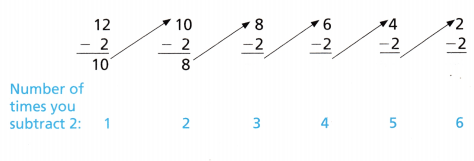Since you subtract 2 six times, there are ___ groups of 2 in 12.
So, Serena and Mandy brought in newspapers for ___ days.
Since you subtract 2 six times, there are 6 groups of 2 in 12.
So, Serena and Mandy brought in newspapers for 6 days.

Be sure to keep subtracting 2 until you are unable to subtract 2 anymore.Read: Twelve divided by two equal six.

Another Way
Count back on a number line.

• Start at 12.
• Count back by 2s as many times as you can. Draw the rest of the jumps on the number line.
• Count the number of times you jumped back 2.You jumped back by 2 six times.
There are ___ jumps of 2 in 12.
12 ÷ 2 = ____
There are 6 jumps of 2 in 12.
12 ÷ 2 = 6
Explanation:
Count back by 2s as many times as you can.
• What do your jumps of 2 represent?
Represents the number of times jumped back 2.

Math Talk
Mathematical Processes

Question 1.
Draw the rest of the jumps on the number line to complete the division equation.
12 ÷ 4 = ___3 jumps
12 ÷ 4 = 3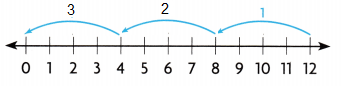Explanation:
Start at 12
Count back by 3s
Count the number of times you jumped back 3.

Math Talk
Mathematical Processes
Explain how counting back on a number line is like using repeated subtraction.
Repeated Subtraction is a method that subtracts the equal number of items from a group, also known as division

Write a division equation.

Question 2.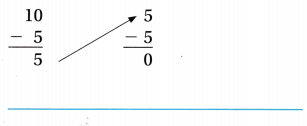10 ÷ 5 = 2Explanation:
Start at 10
Count back by 5s
Count the number of times you jumped back 2.

Question 3.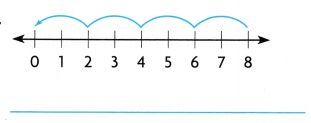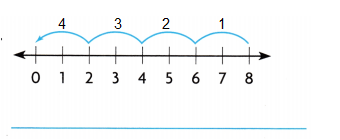8 ÷ 2 = 4
Explanation: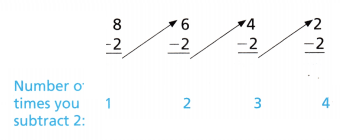Subtract 2 until you reach 0.
Count the number times you subtract 2.

Problem Solving

Question 4.
Matt puts his box tops in 2 equal piles. How many box tops are in each pile?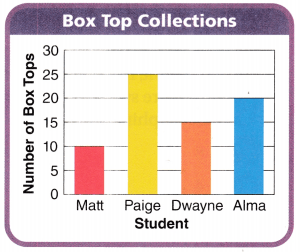5 box tops in each pile.
Explanation:
Matt puts his box tops in 2 equal piles.
Matt has 10 tops,
Number of box tops in each pile
10 ÷ 2 =5

Question 5.
H.O.T. Multi-Step Paige brought an equal number of box tops to school each day for 5 days. Alma also brought an equal number of box tops each day for 5 days. How many box tops did the two students bring in altogether each day? Explain.9 box tops each day.
Explanation:
Paige brought an equal number of box tops to school each day for 5 days.
Total box tops Paige have 25
So, 25 ÷ 5 = 5
Alma also brought an equal number of box tops each day for 5 days.
Total box tops Alma have 20
20 ÷ 5 = 4
Number of box tops the two students brought altogether each day
5 + 4 = 9

Question 6.
Write Math what’s the Question? Dwayne puts all his box tops into bins. He puts an equal number in each bin. The answer is 5. What’s the question?
3 equal number of box tops in each bin.
Explanation:
Dwayne has 15 box tops.
the answer is given as 5
So, 15 ÷ 5 = 3
He puts 3 equal number of box tops in each bin.

Question 7.
H.O.T. Pose a Problem Write a word problem about collecting box tops that can be solved by using the division equation 28 ÷ 7 = 4. Then solve the problem.
Paige adds 3 more box tops and puts in 7 equal piles. How many box tops are in each box?
Each box has 4 box tops.
Explanation:
Paige have 25 box tops
Paige adds 3 more box tops and puts in 7 equal piles.
Total box tops in each box
28 ÷ 7 = 4.
Each box has 4 box tops.

Fill in the bubble for the correct answer choice.
You may use models or strategies to solve.

Question 8.
Multi-Step There are 16 dolphins in a pod. Each pod has the same number of males and females. The female dolphins are swimming in pairs. How many pairs of female dolphins are there?
(A) 8
(B) 4
(C) 6
(D) 2
Option(B)
Explanation:
There are 16 dolphins in a pod.
Each pod has the same number of males and females 16 ÷ 8 = 2
So, 8 males and 8 females.
The female dolphins are swimming in pairs.
Total pairs of female dolphins
8 ÷ 2 = 4

Question 9.
Representations What division equation is shown?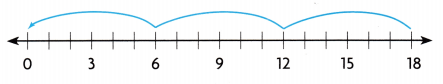(A) 18 ÷ 2 = 9
(B) 18 ÷ 9 = 2
(C) 2 × 9 = 18
(D) 18 ÷ 6 = 3
Option(D)
Explanation:
Given number line is divided into 3 equal parts.
Each part contains 6 counters.

Question 10.
Multi-Step Tim has 30 grapes. He keeps 9 grapes for himself. Then he gives 7 grapes each to some of his friends. To how many friends does Tim give grapes?
(A) 3
(B) 4
(C) 10
(D) 7
Option(A)
Explanation:
Tim has 30 grapes.
He keeps 9 grapes for himself.
So, 30 – 9 = 21
Then he gives 7 grapes each to some of his friends.
Tim gives grapes to his friends
21 ÷ 7 = 3

Texas Test Prep

Question 11.
Renee has 18 photos. She wants to put the same number of photos on each of 6 pages in her photo album. How many photos will be on each page?
(A) 4
(B) 3
(C) 2
(D) 5
Option(B)
Explanation:
Renee has 18 photos.
She wants to put the same number of photos on each of 6 pages in her photo album.
Number of photos on each page
18 ÷ 6 = 3

### Texas Go Math Grade 3 Lesson 10.4 Homework and Practice Answer Key

Write a division equation.

Question 1.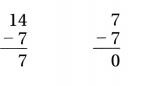14 ÷  7 = 2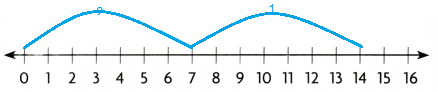Explanation:
Start at 14
Count back by 7s
Count the number of times you jumped back 2.

Question 2.9 ÷ 3 = 3
Explanation:
Given number line is divided into 3 equal parts.
Each part contains 3 counters.

Problem Solving

Use the graph for 3-6.

Question 3.
Beth sorts an equal number of her bottles into 3 bins. How many bottles are in each bin?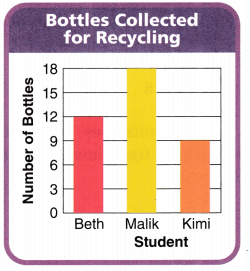4 bottles in each bin.
Explanation:
Beth sorts an equal number of her bottles into 3 bins.
Total number of bottles of Beth 12
Number of bottles in each bin
12 ÷ 3 = 4

Question 4.
Malik and Kimi combine their bottles, then sort an equal number of each into 3 bins. How many bottles are in each bin?
9 bottles in each bin.
Explanation:
Malik and Kimi combine their bottles, 18 + 9 = 27
then sort an equal number of each into 3 bins.
Number of bottles in each bin
27 ÷  3 = 9

Question 5.
Kimi collects another 7 bottles and sorts an equal number into 4 bins. How many bottles are in each bin?
4 bottles in each bin.
Explanation:
Kimi has 9 bottles at first and then,
collects another 7 bottles and sorts an equal number into 4 bins.
Number of bottles in each bin
16 ÷ 4 = 4

Question 6.
All of the students want to combine their bottles and sort an equal number into 6 bins. Can they do this? Explain.
No, they cannot sort an equal number into 6 bins.
Explanation:
All of the students want to combine their bottles and sort an equal number into 6 bins.
Total number of bottles altogether 12 + 18 + 9 = 39
No, they cannot sort an equal number into 6 bins,
because when 39 ÷ 6, we get remainder as 3.

Texas test Prep

Lesson Check

Question 7.
Wendy draws this number line.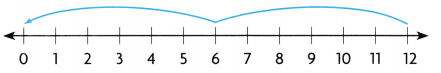Which division equation does she show?
(A) 12 ÷ 6 = 2
(B) 2 × 6 = 12
(C) 12 ÷ 3 = 4
(D) 12 ÷ 4 = 3
Option(A)
Explanation:
Division using number line diagrams with one-unit intervals,
indicate hops in the reverse order as you divide up to 12 using 1 to 10 as divisors, and complete the
division sentences.

Question 8.
Tomas draws this number line.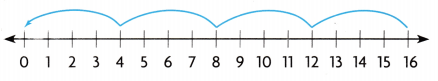Which division equation does he show?
(A) 16 ÷ 8 = 2
(B) 16 ÷ 2 = 8
(C) 4 × 4 = 16
(D) 16 ÷ 4 = 4
Option(D)
Explanation:
The number line has 4 equal parts, in 4 groups.
Total numbers on number line are 16.

Question 9.
Key bakes 18 mini-pies. There are 6 pies on each tray. How many trays does Key use?
(A) 12
(B) 6
(C) 4
(D) 3
Option(D)
Explanation:
Key bakes 18 mini-pies.
There are 6 pies on each tray.
Number of trays Key used
18 ÷ 6 = 3

Question 10.
Keesha has 14 shoes in all. How many pairs of shoes does Keesha have?
(A) 7
(B) 2
(C) 28
(D) 14
Option(A)
Explanation:
Keesha has 14 shoes in all.
Total pairs of shoes Keesha have
14 ÷ 2 = 7

Question 11.
Multi-Step Tara has 32 beads. She takes out 4 red heads, then sorts the rest into 4 equal groups. How many beads are in each group?
(A) 7
(B) 4
(C) 8
(D) 9
Option (A)
Explanation:
She takes out 4 red beads,
so, 32 – 4 = 28
then sorts the rest into 4 equal groups.
Number of beads in each group
28 ÷ 4 = 7

Question 12.
Multi-Step Jeff has a booklet of 30 stickers. He uses one page of stickers. If there are 6 stickers on each page, how many pages were left?
(A) 4
(B) 24
(C) 5
(D) 6